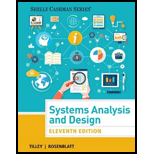Chapter TPC, Problem 5RQSystems Analysis and Design (Shell...

11th Edition
Scott Tilley + 1 other
ISBN: 9781305494602

Solutions

Chapter
SectionSystems Analysis and Design (Shell...

11th Edition
Scott Tilley + 1 other
ISBN: 9781305494602
Textbook Problem

What formula do you use to calculate the payback period?

Program Plan Intro

The formula to calculate payback period.

Program Explanation

Payback period is defined as the total time it will take to expect returns from an investment in form of incomes. Investments with shorter payback period is mostly preferred and are associated with less risk.

To calculate payback period cost of investment and annual cash flow (CF) is considered...

Still sussing out bartleby?

Check out a sample textbook solution.

See a sample solution

The Solution to Your Study Problems

Bartleby provides explanations to thousands of textbook problems written by our experts, many with advanced degrees!

Get Started

Find more solutions based on key concepts